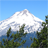# Calculate Geometry - Custom Python Set Units via CSV

457
4
02-08-2021 11:26 AMNew Contributor II

ArcGIS Dektop 10.6.1

I have to repeat a process on many GDBs quarterly. Need to calculate spatial fields (e.g. ccordinateX, coordinateY, featureArea, featureAreaUom, featureLength, featureLengthUom, featurePerimeter, featurePerimeterUom) with units based on an input CSV list of feature classes. Feature classes have spatial unit attributes that may differ based on the input CSV. CSV sample:

 Feature_Class Area UOM_Area Line UOM_Line Grid_A ACRES Acres MILES_US US Survey Mile Ber_L NA NA FEET_US Feet RegA_A SQUARE_YARDS Square Yards FEE_US Feet AirDot_P NA NA NA NA

I merged some existing scripts (I'm still a novice with python) to get the script below, which works (except I have to build out for more fields), except it sets the featureArea and featureLength units to meters based on the coordinate system of the feature classes, rather than taking units from the CSV. There is a commented out line close to the bottom "row[count] = SHAPE.LENGTH@r" that is the area where I think this needs to be altered?

``````import arcpy, os, sys, shutil, csv

Repository = arcpy.GetParameterAsText(0)
InCSV = arcpy.GetParameterAsText(1)

arcpy.env.workspace = Repository

#Create list of feature classes
def getFCList(vPath):
vFullFCList = []
vDSList = arcpy.ListDatasets()
for ds in vDSList:
vFCList = arcpy.ListFeatureClasses('*', 'All', ds)
vFullFCList = vFullFCList + vFCList
vFCList2 = arcpy.ListFeatureClasses()
vFullFCList = vFullFCList + vFCList2
return vFullFCList

#Get list of feature classes
fcList = getFCList(arcpy.env.workspace)
#List of fields to be checked
geoFields = [ "coordinateX", "coordinateY", "featureLength", "featureLengthUom", "featureArea", "featureAreaUom", "featurePerimeter", "featurePerimeterUom"]

for fc in fcList:
fields = []
desc = arcpy.Describe(fc)
dataSet = desc.path
fieldList = arcpy.ListFields(fc)
#If the following fields exist, adds the field name to a list to be used later.
for field in fieldList:
if field.name == "coordinateX":
fields.append(field.name)
fields.append("SHAPE@X")
elif field.name == "coordinateY":
fields.append(field.name)
fields.append("SHAPE@Y")
elif field.name == "featureLength":
fields.append(field.name)
fields.append("SHAPE@LENGTH")
elif field.name == "featureArea":
fields.append(field.name)
fields.append("SHAPE@AREA")
elif field.name == "featurePerimeter":
fields.append(field.name)
fields.append("SHAPE@LENGTH")
else:
fields.append(field.name)

#The tool checks if the field exists within a feature class
#If the field exists it will populate that field with its corresponding value
with open(InCSV, 'r') as source:
UpdateName = desc.name
for r in rdr:
if len(fields) > 0:
with arcpy.da.UpdateCursor(fc, fields) as cursor:
for row in cursor:
count = 0
while count < len(fields):
if fields[count] == "coordinateX":
idx = fields.index("SHAPE@X")
row[count] = row[idx]
elif fields[count] == "featureLength" and UpdateName == r:
idx = fields.index("SHAPE@LENGTH")
row[count] = row[idx]
#row[count] = SHAPE.LENGTH@r
elif fields[count] == "featureAreaUom" and UpdateName == r:
row[count] = r
elif fields[count] == "featureLengthUom" and UpdateName == r:
row[count] = r
count += 1

cursor.updateRow(row)
sys.exit()``````

Tags (5)
4 RepliesbyMVP Frequent Contributor

Personally I would find it simpler to pass these into an existing GP tool such as Calculate Geometry Attributes (Data Management)—ArcGIS Pro | Documentation

You could just loop through the for r in rdr, then use r, r... etc as arguments to the tool.New Contributor II

Thanks. Probably beyond my ability and time, but I see what I can do with this if something else doesn't come up.byMVP Frequent Contributor

Your csv is well set up for this, this is an example of the process:

``````with open(InCSV, 'r') as source:
for r in rdr:
fc_name = r
area_unit = r
length_unit = r
geometry_fields = []
if area_unit != 'NA':
geometry_fields.append('AREA')
if length_unit != 'NA':
geometry_fields.append('LENGTH')by